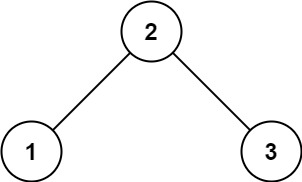## 98. Validate Binary Search Tree

Given the `root` of a binary tree, determine if it is a valid binary search tree (BST).

A valid BST is defined as follows:

• The left subtree of a node contains only nodes with keys less than the node's key.
• The right subtree of a node contains only nodes with keys greater than the node's key.
• Both the left and right subtrees must also be binary search trees.

Example 1:```Input: root = [2,1,3]
Output: true
```

Example 2:```Input: root = [5,1,4,null,null,3,6]
Output: false
Explanation: The root node's value is 5 but its right child's value is 4.
```

Constraints:

• The number of nodes in the tree is in the range `[1, 104]`.
• `-231 <= Node.val <= 231 - 1`

## Rust Solution

``````struct Solution;
use rustgym_util::*;

trait Inorder {
fn inorder(&self, visit: &mut dyn FnMut(i32));
}

fn inorder(&self, visit: &mut dyn FnMut(i32)) {
if let Some(node) = self {
let node = node.borrow();
Self::inorder(&node.left, visit);
visit(node.val);
Self::inorder(&node.right, visit);
}
}
}

impl Solution {
fn is_valid_bst(root: TreeLink) -> bool {
let mut prev: Option<i32> = None;
let mut res = true;
root.inorder(&mut |x| {
if let Some(y) = prev {
if x <= y {
res = false;
}
}
prev = Some(x);
});
res
}
}

#[test]
fn test() {
let root = tree!(2, tree!(1), tree!(3));
let res = true;
assert_eq!(Solution::is_valid_bst(root), res);
let root = tree!(5, tree!(1), tree!(4, tree!(3), tree!(6)));
let res = false;
assert_eq!(Solution::is_valid_bst(root), res);
}
``````

Having problems with this solution? Click here to submit an issue on github.